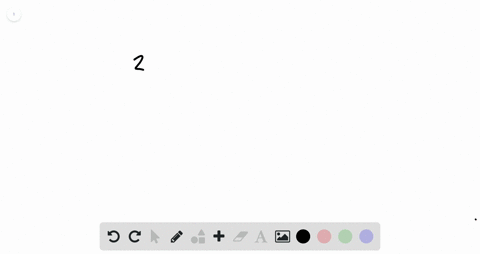Enroll in one of our FREE online STEM summer camps. Space is limited so join now!View Summer Courses### For Exercises $1-12,$ determine whether the order…

00:47California University of Pennsylvania

Need more help? Fill out this quick form to get professional live tutoring.

Get live tutoring
Problem 2

For Exercises $1-12,$ determine whether the ordered pair is a solution for the equation.
$$(3,1) ; 2 x-y=5$$

yes

## Discussion

You must be signed in to discuss.

## Video Transcript

is the solution? 31 A solution to the equation. Two X minus, like was five. So what we're going to do is take our ordered pair and we're going to replace the X and the lie with our ordered pair. So we're gonna pluck three in for X. Someone in for Why? So we have two times three minus one equals five calling. Order of operations. Two times three is six minus one equals five six minus one is five since five equals five, then the ordered pair 31 is a solution to the equation. Two x minus Y equals five.